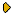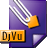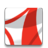Журнал Экспериментальной и Теоретической Физики
 НАЧАЛО | ПОИСК | ДЛЯ АВТОРОВ | ПОМОЩЬОбщая информация о журналеЗолотые страницыАдреса редакцииСодержание журналаСообщения редакцииПравила для авторовЗагрузить статьюПроверить статус статьиЖЭТФ, Том 119, Вып. 6, стр. 1257 (Июнь 2001)
(Английский перевод - JETP, Vol. 92, No 6, p. 1090, June 2001 доступен on-line на www.springer.com )

THE INFLUENCE OF THE CHEMICAL POTENTIAL OSCILLATIONS ON THE DE HAAS-VAN ALPHEN EFFECT IN QUASI-TWO-DIMENSIONAL COMPOUNDS

Поступила в редакцию: 16 Января 2001

PACS: 71.18.+yDJVU (57.5K)PDF (223.6K)

The de Haas-van Alphen effect in quasi-two-dimensional metals is studied at arbitrary parameters. Oscillations of the chemical potential can substantially change the temperature dependence of harmonic amplitudes that is commonly used to determine the effective electron mass. The processing of the experimental data using the standard Lifshitz-Kosevich formula can therefore lead to substantial errors even in the strong harmonic damping limit. This may explain the difference between the effective electron masses determined from the de Haas-van Alphen effect and the cyclotron resonance measurements. The oscillations of the chemical potential and the deviations from the Lifshitz-Kosevich formula depend on the reservoir density of states that exists in organic metals due to open sheets of the Fermi surface. This dependence can be used to determine the density of electron states on open sheets of the Fermi surface. We present analytical results of the calculations of harmonic amplitudes in some limiting cases that show the importance of the chemical potential oscillations. We also describe a simple algorithm for a numerical calculation of the harmonic amplitudes for arbitrary reservoir density of states, arbitrary warping, spin-splitting, temperature, and Dingle temperature.

Сообщить о технических проблемах